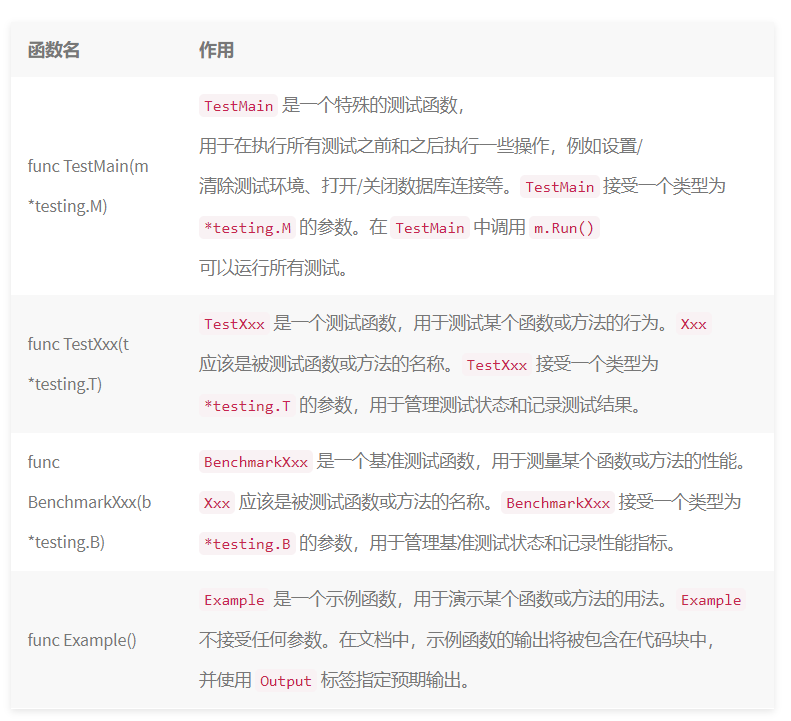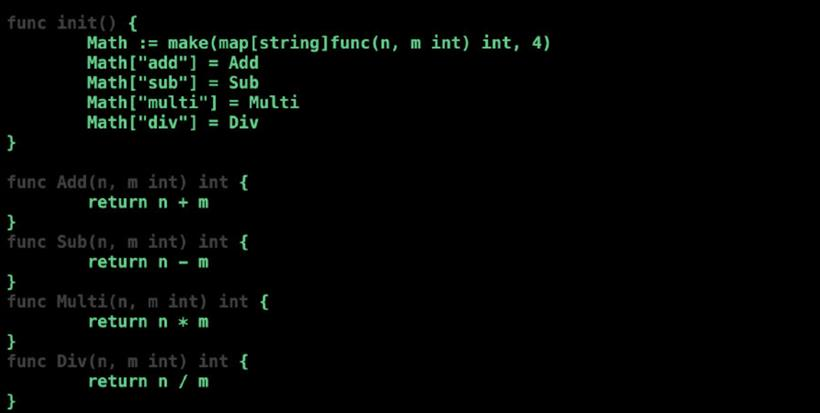• Go开发的常用工具汇总
• 发布于 1个月前
• 49 热度
0 评论
Go 语言目前在云原生和服务端中间件开发领域方兴未艾，相比 Java 的在服务端和大数据应用规模还是相差疏远，Go 目前应用得益于容器技术和容器编排机器的普及，还有服务端开发越来越流行的分布式微服务架构。我个人接触 Go 语言是从 2019 年开始已经有 4 年多了，Go 入门简单编译速度相比 C 和 C++ 、Rust 要快得多，但是要深入掌握 Go 语言和 Go 运行时就要花费些时间自己去研究 Go 运行时的源代码，Go 开发相关的工具链也很完善，例如单元测试 、性能测试 、程序性能分析 pprof 、 交叉构建工具等，这片博文我将会介绍 Go 相关的工具链使用。

Go 作为一个静态编译语言生成的二进制为相应平台的架构指令，这就带来一个新的问题如何做交叉编译，并且每个平台对应系统调用 API 接口可能不同或者实现也不同，需要在构建二进制文件的时候过滤掉一些源代码文件，Go 官方提供 build 标签能帮助开发者来实现此功能。

```// +build ignore
package main

import "fmt"

func main() {
num := 3601
fmt.Println(toTime(num))
}

func toTime(num int) string {
return fmt.Sprintf("%d = %dh:%dm:%ds", num, (num / 3600), (num / 3600 / 60), (num % 60))
}```

```// +build linux,amd64 && !darwin && !386 && !arm64
package main
import "syscall"

func main() {
syscall.Syscall(1, 0, 0, 0) // This is the "exit" syscall on Linux
}```

```package main

import "fmt"

//go:generate sh -c "echo \"package main\n\nconst VERSION = \\\"\$(go version | awk '{print \$3}')\\\"\" > version.go"

func main() {
fmt.Println("Version:", VERSION)
}```

```type People struct {
Name string `json:"name"`
Age  int    `json:"age"`
} // +person

//go:generate some-code-generator --name=ToString --group=toStr
func ToString() string {
// dosomething
}```

//go:cgo_import_dynamic funcName library.funcName "/path/mylib.so"

```package main

import (
"unsafe"
)

//go:noescape
func Copy(dest, src []byte) int {
n := len(src)
if len(dest) < n {
n = len(dest)
}
if n > 0 {
copy(dest[:n], src[:n])
}
return n
}

func main() {
buf1 := make([]byte, 10)
buf2 := make([]byte, 20)

// 使用 Go 语言的 copy 函数复制切片
n := copy(buf1, buf2)
println(n)

// 使用 C 语言的 memcpy 函数复制内存
n = Copy(buf1, buf2)
println(n)

// 使用 unsafe 包将一个字符串转换为一个不可变的 []byte 切片
s := "hello"
b := *(*[]byte)(unsafe.Pointer(&s))
println(string(b))
}```

```package main

import "fmt"

//go:noinline
func myFunc(x int) int {
return x + 1
}

func main() {
// 调用 myFunc 函数 10 次，并打印返回值
for i := 0; i < 10; i++ {
fmt.Println(myFunc(i))
}
}``````package main

import (
"fmt"
"os"
"testing"
)

func TestMain(m *testing.M) {
// 执行一些初始化操作，例如设置测试环境
fmt.Println("TestMain setup")
// 调用 m.Run() 运行所有测试
exitCode := m.Run()
// 执行一些清理操作，例如关闭数据库连接
fmt.Println("TestMain teardown")
// 退出测试，并使用 exitCode 作为退出状态码
os.Exit(exitCode)
}

// 测试加法函数的行为
if result != 5 {
t.Errorf("Expected 5, but got %d", result)
}
}

func add(a, b int) int {
return a + b
}```

```# go test -run=^TestAdditio\$
if result != 5 {
t.Errorf("Addition failed. Expected 5 but got %d", result)
}
}```

```package main

import (
"math/rand"
"testing"
)

// 基准测试随机数生成器的性能 go test -bench=.
func BenchmarkRand(b *testing.B) {
for i := 0; i < b.N; i++ {
rand.Int()
}
}```

```func TestSum(t *testing.T) {
type test struct {
name     string
numbers  []int
expected int
}

tests := []test{
{name: "Test Sum Positive Numbers", numbers: []int{1, 2, 3, 4, 5}, expected: 15},
{name: "Test Sum Negative Numbers", numbers: []int{-1, -2, -3, -4, -5}, expected: -15},
{name: "Test Sum Mixed Numbers", numbers: []int{-1, 2, 3, -4, 5}, expected: 5},
}

for _, tc := range tests {
t.Run(tc.name, func(t *testing.T) {
result := Sum(tc.numbers...)
if result != tc.expected {
t.Errorf("Sum(%v) = %d; want %d", tc.numbers, result, tc.expected)
}
})
}
}```

Go 语言在设计的时候就借鉴过 Java 的设计，Go 作为一个工业工程化的编程语言也提供类似于 Java Doc 工具，但是没有 Java Doc 功能那么强大，可以满足日常开发使用，想要自动生成代码的文档，只需要安装 go-lint 相关工具将可以帮助提示相关注释规范，常用注释格式如下：
```// Package mypack provides utility functions for performing various operations.
package mypack

// MaxRetryCount specifies the maximum number of times to retry a failed operation.
var MaxRetryCount = 5

// IsZero returns true if the complex number is zero.
func (c Complex) IsZero() bool {
return c.real == 0 && c.imag == 0
}```

```package calculator

import (
"fmt"
"os"
)

// Add returns the sum of two integers.
func Add(a, b int) int {
return a + b
}

// Output: 5
}

func main() {
doc := `Package calculator provides basic math operations.`
fmt.Fprintln(os.Stdout, doc)
}```

```package math

func init() {
Math := make(map[string]func(n, m int) int, 4)
Math["sub"] = Sub
Math["multi"] = Multi
Math["div"] = Div
}

func Add(n, m int) int {
return n + m
}
func Sub(n, m int) int {
return n - m
}
func Multi(n, m int) int {
return n * m
}
func Div(n, m int) int {
return n / m
}```

```package math_test

import "testing"

func TestMath(t *testing.T) {
// 自定义测试结构体
type MathCase struct {
n, m, result int
}
// 自定义子测试map
testGroup := map[string]MathCase{
"sub":   {3, 1, 2},
"multi": {3, 2, 6},
"div":   {6, 2, 3},
}
// 测试执行函数
for name, mathCase := range testGroup {
t.Run(name, func(t *testing.T) {
s := -1
switch name {
case "sub":
s = Sub(mathCase.n, mathCase.m)
case "multi":
s = Multi(mathCase.n, mathCase.m)
case "div":
s = Div(mathCase.n, mathCase.m)
default:
t.Fatalf("No executable testing name :%s", name)
}
if mathCase.result != s {
t.Fatalf(" add computer result error， want %d , got %d", mathCase.result, s)
}
})
}
}```Go 语言中还提供基于程序 runtime 和内存性能分析工具 pprof ，可以在程序运行的时候分析程序的运行状态信息内存占用情况，对内存进行分析采样数据，最后生成对应数据报表信息供开发者人员查找程序问题，支持的模式有命令行模式，还有 web 界面模式，报告生成模式，用的最多还是命令模式和报告生成模式。

```package main

import (
"log"
"net/http"
_ "net/http/pprof"
)

func main() {
// 在主程序的某处开启 pprof 监听，端口号为 6060
log.Println("start pprof server...")
go func() {
err := http.ListenAndServe("localhost:6060", nil)
if err != nil {
log.Fatalln("pprof server start failed: ", err)
}
}()

// 程序的其他逻辑代码
...
}```

```package main

import (
"fmt"
"os"
"runtime/pprof"
)

func main() {
// ...
// 堆代码 duidaima.com
// 创建一个 CPU profile 文件
f, err := os.Create("cpu.prof")
if err != nil {
panic(err)
}
defer f.Close()

// 开始 CPU profiling
if err := pprof.StartCPUProfile(f); err != nil {
panic(err)
}
defer pprof.StopCPUProfile()

// 需要分析的代码
for i := 0; i < 100000; i++ {
fmt.Println(i)
}

// ...
}```

```package main

import (
"flag"
"fmt"
"os"
"runtime/pprof"
"time"
)

// 一段有问题的代码
func logicCode() {
var c chan int
for {
select {
case v := <-c:
fmt.Printf("recv from chan, value:%v\n", v)
default:

}
}
}

func main() {
var isCPUPprof bool
var isMemPprof bool

flag.BoolVar(&isCPUPprof, "cpu", false, "turn cpu pprof on")
flag.BoolVar(&isMemPprof, "mem", false, "turn mem pprof on")
flag.Parse()

if isCPUPprof {
file, err := os.Create("./cpu.pprof")
if err != nil {
fmt.Printf("create cpu pprof failed, err:%v\n", err)
return
}
pprof.StartCPUProfile(file)
defer pprof.StopCPUProfile()
}
for i := 0; i < 8; i++ {
go logicCode()
}
time.Sleep(20 * time.Second)
if isMemPprof {
file, err := os.Create("./mem.pprof")
if err != nil {
fmt.Printf("create mem pprof failed, err:%v\n", err)
return
}
pprof.WriteHeapProfile(file)
file.Close()
}
}```

```\$: go tool pprof cpu.pprof
Type: cpu
Time: May 16, 2020 at 12:59pm (CST)
Duration: 20.14s, Total samples = 56.90s (282.48%)
Entering interactive mode (type "help" for commands, "o" for options)
(pprof) top3
Showing nodes accounting for 51.91s, 91.23% of 56.90s total
Dropped 7 nodes (cum <= 0.28s)
Showing top 3 nodes out of 4
flat  flat%   sum%        cum   cum%
23.04s 40.49% 40.49%     45.18s 79.40%  runtime.selectnbrecv
17.26s 30.33% 70.83%     18.93s 33.27%  runtime.chanrecv
11.61s 20.40% 91.23%     56.83s 99.88%  main.logicCode
(pprof) list logicCode
Total: 56.90s
ROUTINE ======================== main.logicCode in /Users/ding/Documents/GO_CODE_DEV/src/Lets_Go/lets_37_pprof/main.go
11.61s     56.83s (flat, cum) 99.88% of Total
.          .     16:// 一段有问题的代码
.          .     17:func logicCode() {
.          .     18:   var c chan int
.          .     19:   for {
.          .     20:           select {
11.61s     56.83s     21:           case v := <-c:
.          .     22:                   fmt.Printf("recv from chan, value:%v\n", v)
.          .     23:           default:
.          .     24:
.          .     25:           }
.          .     26:   }
(pprof) ```